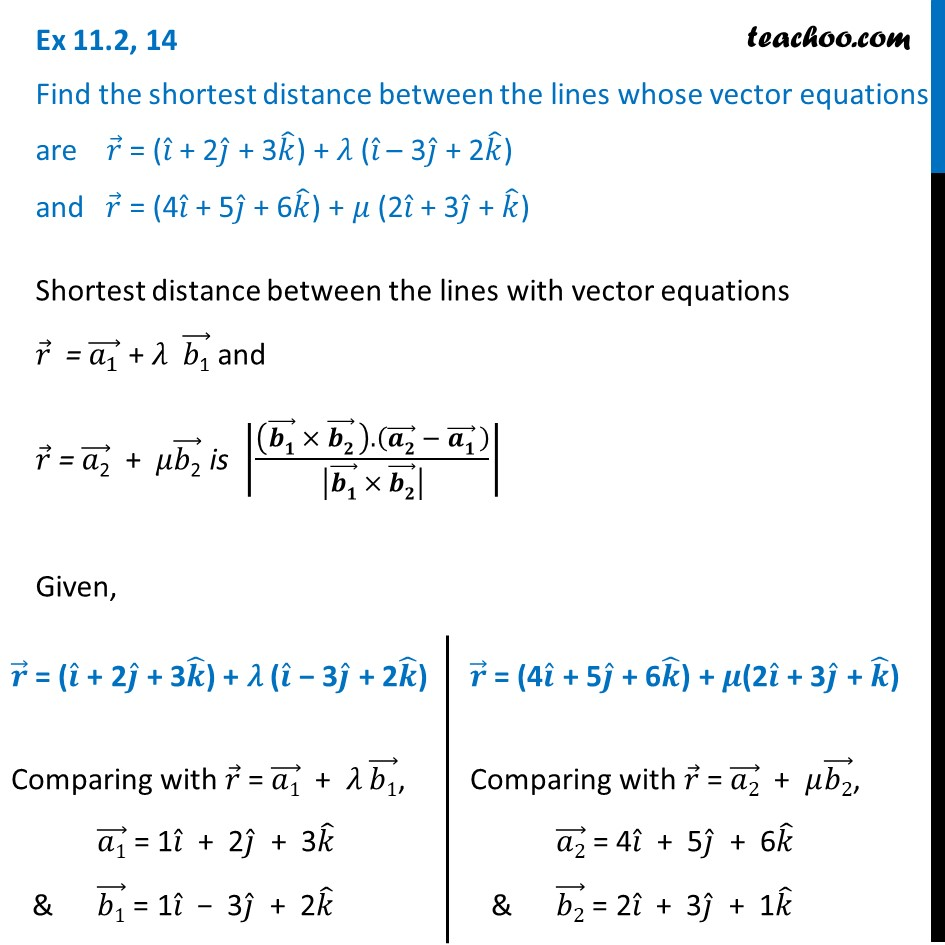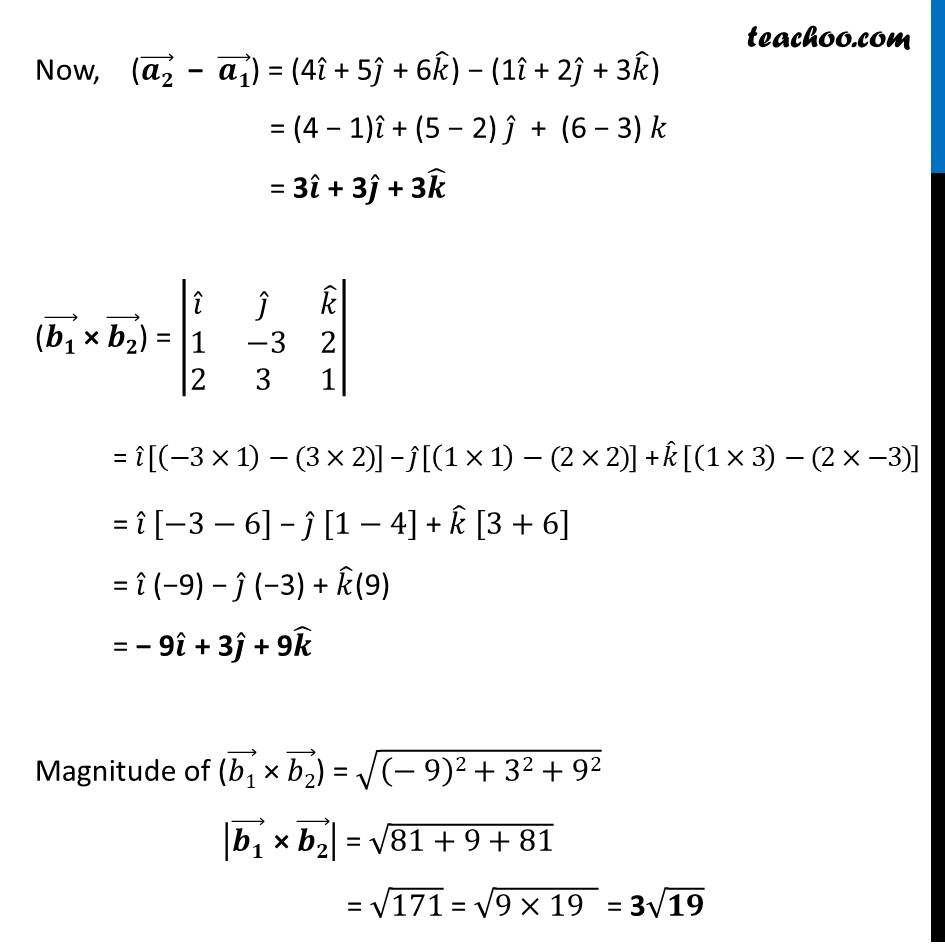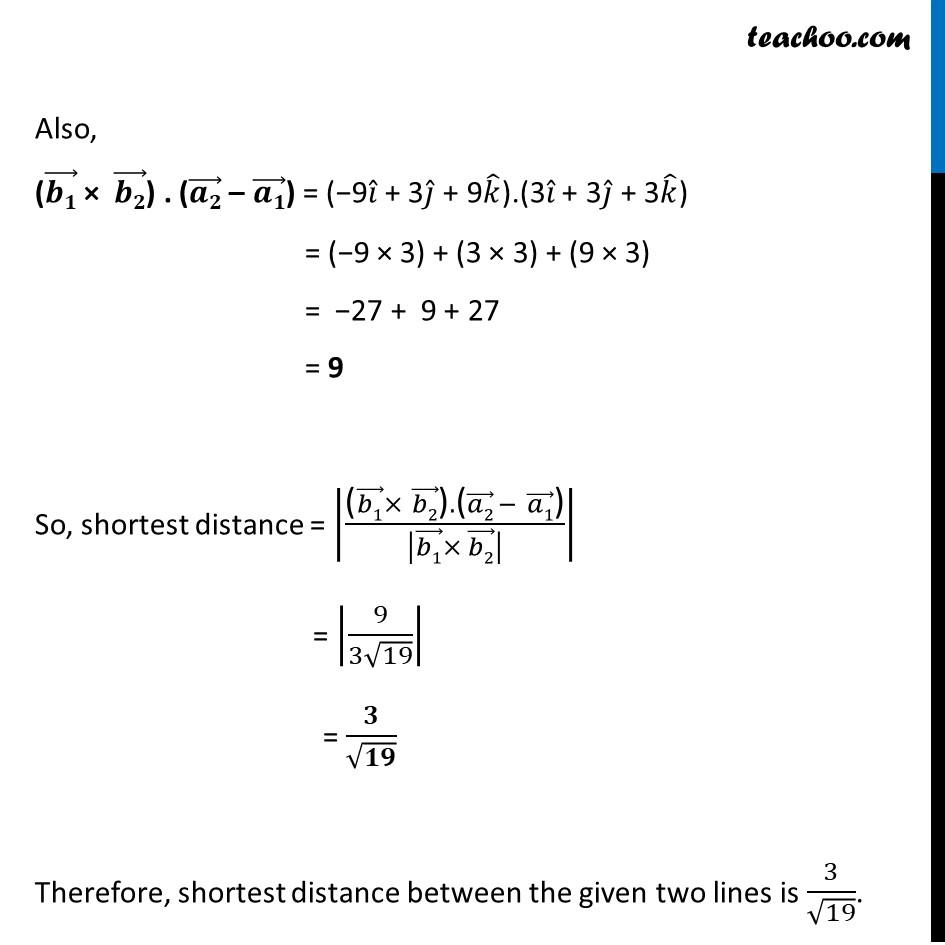Ex 11.2

Chapter 11 Class 12 Three Dimensional Geometry
Serial order wiseLearn in your speed, with individual attention - Teachoo Maths 1-on-1 Class

### Transcript

Ex 11.2, 14 Find the shortest distance between the lines whose vector equations are 𝑟 ⃗ = (𝑖 ̂ + 2𝑗 ̂ + 3𝑘 ̂) + 𝜆 (𝑖 ̂ – 3𝑗 ̂ + 2𝑘 ̂) and 𝑟 ⃗ = (4𝑖 ̂ + 5𝑗 ̂ + 6𝑘 ̂) + 𝜇 (2𝑖 ̂ + 3𝑗 ̂ + 𝑘 ̂)Shortest distance between the lines with vector equations 𝑟 ⃗ = (𝑎_1 ) ⃗ + 𝜆 (𝑏"1" ) ⃗ and 𝑟 ⃗ = (𝑎"2" ) ⃗ + 𝜇(𝑏"2" ) ⃗ is |(((𝒃𝟏) ⃗ × (𝒃𝟐) ⃗ ).((𝒂𝟐) ⃗ − (𝒂𝟏) ⃗ ))/|(𝒃𝟏) ⃗ × (𝒃𝟐) ⃗ | | Given, 𝒓 ⃗ = (𝒊 ̂ + 2𝒋 ̂ + 3𝒌 ̂) + 𝜆 (𝒊 ̂ − 3𝒋 ̂ + 2𝒌 ̂) Comparing with 𝑟 ⃗ = (𝑎1) ⃗ + 𝜆 (𝑏1) ⃗, (𝑎1) ⃗ = 1𝑖 ̂ + 2𝑗 ̂ + 3𝑘 ̂ & (𝑏1) ⃗ = 1𝑖 ̂ − 3𝑗 ̂ + 2𝑘 ̂ 𝒓 ⃗ = (4𝒊 ̂ + 5𝒋 ̂ + 6𝒌 ̂) + 𝝁(2𝒊 ̂ + 3𝒋 ̂ + 𝒌 ̂) Comparing with 𝑟 ⃗ = (𝑎2) ⃗ + 𝜇(𝑏2) ⃗, (𝑎2) ⃗ = 4𝑖 ̂ + 5𝑗 ̂ + 6𝑘 ̂ & (𝑏2) ⃗ = 2𝑖 ̂ + 3𝑗 ̂ + 1𝑘 ̂ Now, ((𝒂𝟐) ⃗ − (𝒂𝟏) ⃗) = (4𝑖 ̂ + 5𝑗 ̂ + 6𝑘 ̂) − (1𝑖 ̂ + 2𝑗 ̂ + 3𝑘 ̂) = (4 − 1)𝑖 ̂ + (5 − 2) 𝑗 ̂ + (6 − 3) 𝑘 = 3𝒊 ̂ + 3𝒋 ̂ + 3𝒌 ̂ ((𝒃𝟏) ⃗ × (𝒃𝟐) ⃗) = |■8(𝑖 ̂&𝑗 ̂&𝑘 ̂@1& −3&2@2&3&1)| = 𝑖 ̂ [(−3×1)−(3×2)] − 𝑗 ̂ [(1×1)−(2×2)] + 𝑘 ̂ [(1×3)−(2×−3)] = 𝑖 ̂ [−3−6] − 𝑗 ̂ [1−4] + 𝑘 ̂ [3+6] = 𝑖 ̂ (−9) − 𝑗 ̂ (−3) + 𝑘 ̂(9) = − 9𝒊 ̂ + 3𝒋 ̂ + 9𝒌 ̂ Magnitude of ((𝑏1) ⃗ × (𝑏2) ⃗) = √((− 9)2+32+92) |(𝒃𝟏) ⃗" × " (𝒃𝟐) ⃗ | = √(81+9+81) = √171 = √(9×19 ) = 3√𝟏𝟗 Also, ((𝒃𝟏) ⃗ × (𝒃𝟐) ⃗) . ((𝒂𝟐) ⃗ – (𝒂𝟏) ⃗) = (−9𝑖 ̂ + 3𝑗 ̂ + 9𝑘 ̂).(3𝑖 ̂ + 3𝑗 ̂ + 3𝑘 ̂) = (−9 × 3) + (3 × 3) + (9 × 3) = −27 + 9 + 27 = 9 So, shortest distance = |("(" (𝑏1) ⃗×" " (𝑏2) ⃗")" ."(" (𝑎2) ⃗ −" " (𝑎1) ⃗")" )/|(𝑏1) ⃗× (𝑏2) ⃗ | | = |9/(3√19)| = 𝟑/√𝟏𝟗 Therefore, shortest distance between the given two lines is 3/√19.